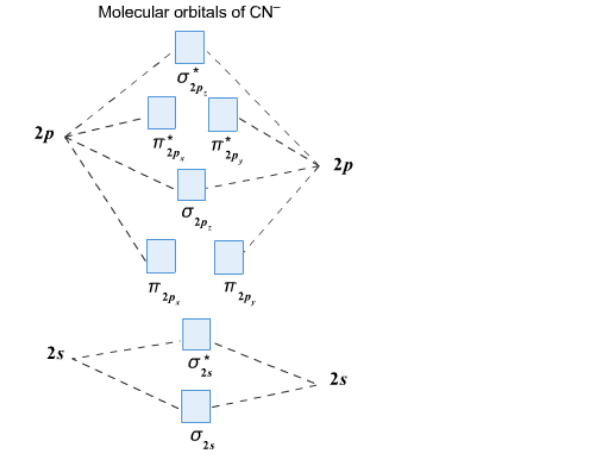# Problem: Complete this molecular orbital diagram for CN– then determine the bond order. Note that the 1s orbital is not shown in this problem.

###### FREE Expert Solution
83% (108 ratings)
###### Problem Details

Complete this molecular orbital diagram for CN then determine the bond order. Note that the 1s orbital is not shown in this problem.Frequently Asked Questions

What scientific concept do you need to know in order to solve this problem?

Our tutors have indicated that to solve this problem you will need to apply the MO Theory: Heteronuclear Diatomic Molecules concept. You can view video lessons to learn MO Theory: Heteronuclear Diatomic Molecules. Or if you need more MO Theory: Heteronuclear Diatomic Molecules practice, you can also practice MO Theory: Heteronuclear Diatomic Molecules practice problems.

What is the difficulty of this problem?

Our tutors rated the difficulty ofComplete this molecular orbital diagram for CN– then determi...as medium difficulty.

How long does this problem take to solve?

Our expert Chemistry tutor, Dasha took 3 minutes and 13 seconds to solve this problem. You can follow their steps in the video explanation above.

What professor is this problem relevant for?

Based on our data, we think this problem is relevant for Professor Geissler's class at CAL.